Problems

The exercises contained within this chapter have been designed to give the reader needed review experience in formal manipulation in probabilistic analysis. The following four problems have the dual purpose of continuing such review and providing experience in the modeling of physical situations that typically arise in urban analysis. The reader is also referred to Problem 3.1 for more review on the basic concepts of probability modeling.

2.1 Discrete random variables: a clean sweep A 300-foot-long city block face contains eight 25-foot-long parking spaces, as shown in Figure P2. 1. For convenience, we have numbered the parking spaces consecutively from 1 to 8. Each Tuesday at 8:00 A.M. a street sweeper attempts to clean the entire street. Any cars parked in those spaces inhibit the work of the sweeper and are, accordingly, each given a \$25 fine (parking ticket). We assume that the probability that any given parking space will be occupied by an illegally parked car is p and that the status (full or empty) of each parking space is independent of the status of all other parking spaces.
 a. For any given Tuesday morning, determine the mean and variance of the number of illegally parked cars on this block face. b. Repeat part (a) for the mean and variance of the total dollar value of parking tickets issued.

For each parked car, the sweeper is unable to sweep the 25 feet contained within that car's parking space and, in addition, because of maneuverability problems, it is unable to sweep within 12.5 feet on either side of that car's parking space (we ignore size differences of cars here). For instance, if cars were only parked in spaces 1 and 3, the sweeper would be unable to sweep 100 consecutive feet of the street, starting at x = 37.5 feet (see Figure P2.1).
 c. Determine the probability of a clean sweep of the street (i.e., that no illegally parked cars are present). d. Given that there are exactly two illegally parked cars on the street, determine the conditional pmf for the length (in feet) of street swept. e. Find the unconditional mean length of street swept.

Hint: Avoid "brute-force" methods by arguing as follows: for any "interior" point x, 62.5 < x < 237.5, the probability that x will be swept is (1 - p)2 Why? Then, the expected length of parking space 4 that will be swept is 25(1 - p)2. Continuing this reasoning and including the other "noninterior" points, you should find that the answer is 75 + 50(l - p) + 175(1 - p)2 feet. (This line of reasoning utilizes ideas of coverage that are explained more fully in Chapter 3.)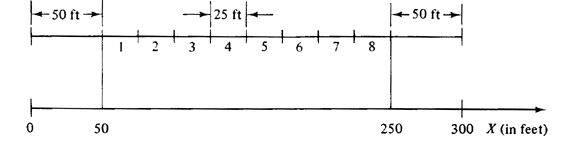2.2 Mixed random variables: a shuttle Suppose that two buses, B1 and B2, are used to shuttle passengers between two stations, S1 and S2, as shown in Figure P2.2. For convenience, we denote the location x1 and x2 of each bus B1 and B2, respectively, by its counterclockwise distance from Sl, 0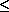x < 2. We are given the following facts about system operation: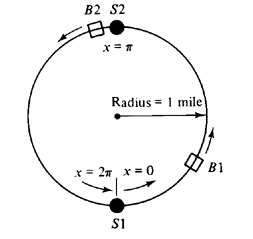1. Statistically, buses B1 and B2 behave identically, as do stations S1 and S2. 2. Each bus spends 25 percent of its time at station Sl, 25 percent at S2, and 50 percent traveling between the two stations. 3. Speed of travel is a fixed constant. a. Draw the cdf and pdf for the location of B1 at a randomly chosen time. [If the cdf is differentiated to yield the pdf, any impulses that result (associated with probability masses) are usually sketched as spikes with numbers adjacent to the spikes equal to the corresponding probability masses.]
For parts (b) and (c), assume that B1 and B2 operate completely independently. (Is that reasonable?)
 b. Sketch and describe the joint pdf for the locations of B1 and B2. (You may have to extend to two dimensions your notion of impulses.) c. Define the headway H between B1 and B2 as the clockwise distance from B1 to B2.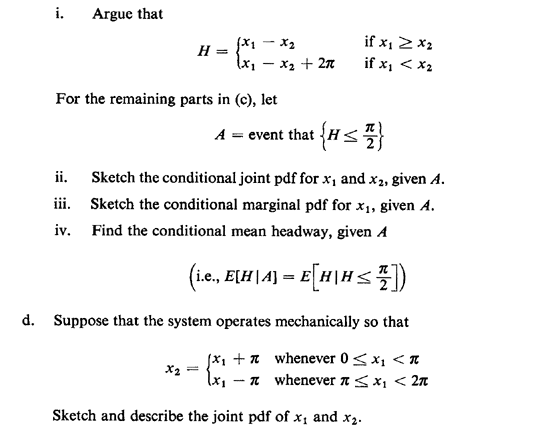2.3 Poisson processes: subways are for waiting. Two one-way subway lines, the A train line and the B train line, intersect at a transfer station, as shown in Figure P2.3. A trains and B trains arrive at the station according to independently operating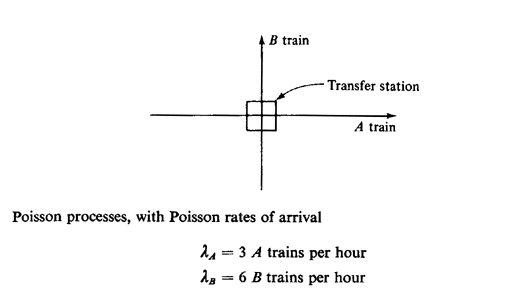We assume that passenger boarding and unboarding occurs almost instantaneously, not unlike true rush-hour conditions in many cities throughout the world!
 a. At a random time, Bart, a prospective passenger, arrives at the station, awaiting an A train. i. What is the pdf for the time he will have to wait? ii. What is the probability that at least 3 B trains will arrive while Bart is waiting? iii. What is the probability that exactly 3 B trains will arrive while Bart is waiting? b. What is the probability that the station handles exactly 9 trains during any given hour? c. If an observer counts the number of trains that the station handles each hour, starting at 8:00 A.M. on Tuesday, what is the expected number of hours until he or she will first count exactly 9 trains during an hour that commences "on the hour"? (e.g., 9: 00 A.M., 10: 00 A.M., 2: 00 P.M.) d. Whenever an A train is ready to depart from the station, it will be held if an approaching B train is within 30 seconds of the station. This delay policy is to facilitate the rapid transfer of passengers from the B train to the A train. i. Approximately what fraction of A trains are delayed in this manner? ii. Given a B-train passenger who benefits from such instantaneous transfer to an A train, using the up-to-30-second-delay policy for the A train, compute his or her mean waiting time reduction and compare to the mean increase in travel time for an already boarded A train passenger. Intuitively, under what conditions would such a delay policy provide net global travel-time reduction?
2.4 Discrete random incidence: school-bus repair Suppose that school buses undergo maintenance only when they break down. For simplicity, we assume that breakdowns occur only at the end of the school day after the driver has driven all the students back to their home neighborhoods. When repairs and other maintenance are completed (requiring at least one day), each school bus behaves as a "new" bus with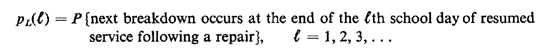Whenever the bus servicing a route has broken down at the end of the previous day, it is replaced by a recently repaired bus that has probability pL(1) of breaking down at the end of its first day back in service, pL(2) of breaking down at the end of its second day, and so on. Each bus has a unique identification number painted boldly on its sides.
 (a) Suppose that on some random day a parent starts accompanying his or her child each day to the school bus stop. Show, by using arguments of random incidence, that the pmf for the number of school days N until the parent sees a different bus on the route (due to a breakdown of the first bus) is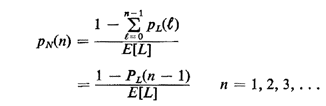Hint: Follow directly the line of reasoning used in developing (2.63)-(2.65). Note that at least one day is required to observe a new bus. This is a case of discrete random incidence, since the random variables involved - the time between events (breakdowns) and the time from random incidence until the next event (N) - can assume only nonnegative integer values. (b) Explain intuitively the result pN(1) = 1/E[L]. (c) Following the conditioning argument used to derive (2.66), show that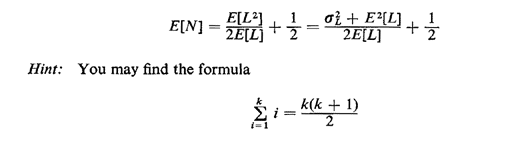useful. Does the addition of the "1/2" term make sense intuitively? Evaluate E[N] assuming that E[L] = 50 days and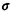L = 30 days.